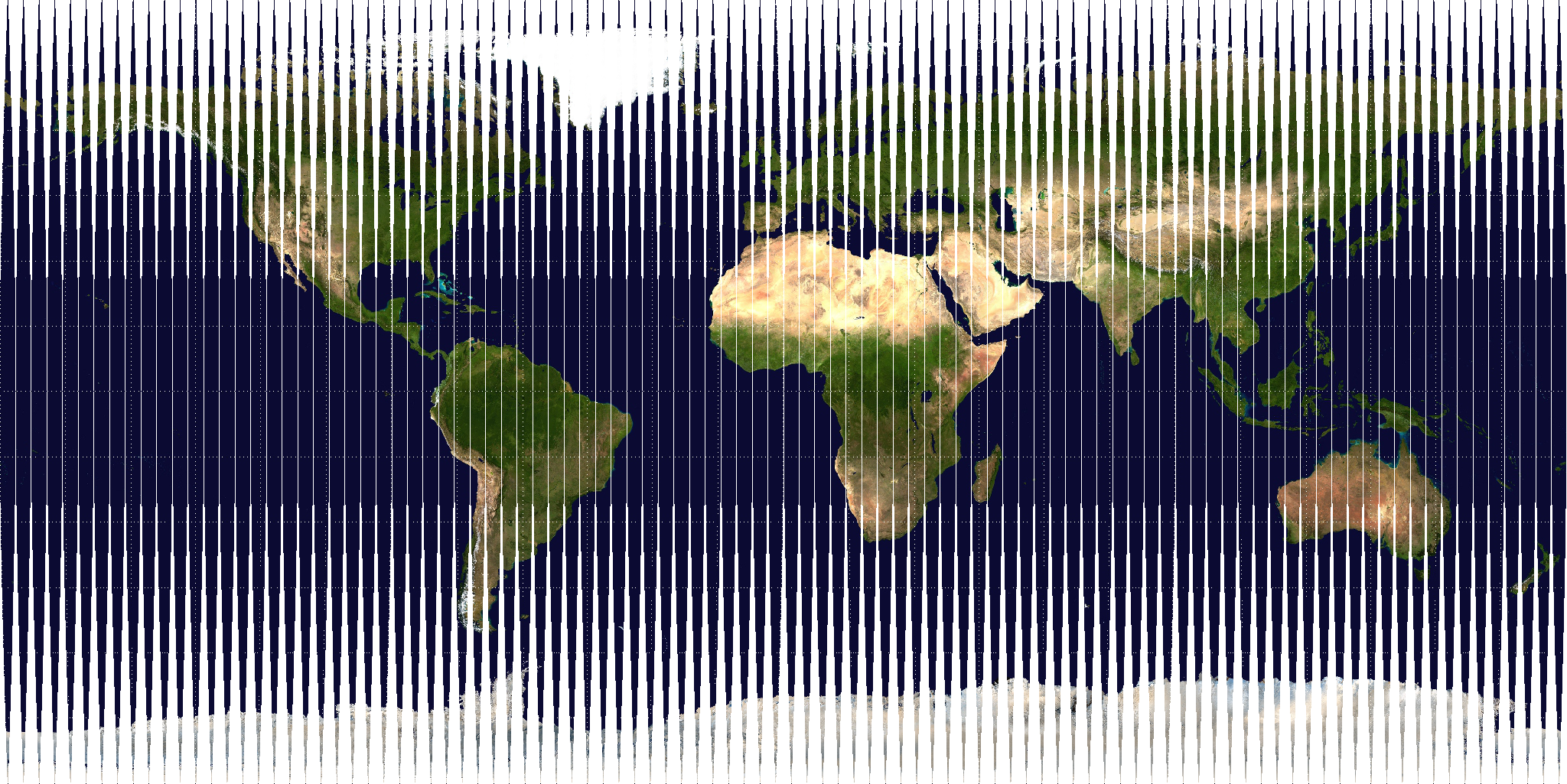# Maps

I like making maps. Here are some that I've made:

## A sinusoidal projection, but with 100 slicesThis map may look like a normal equirectangular projection at first glance, but that is deceit! If you look closely, it is divided into 100 evenly-sized slices. These slices use a sine function to change the x-coordinates in every slice according to their distance from the equator. Using this transformation, the map actually becomes an equal-area projection, meaning that areas are preserved. What I find interesting is that the gut reaction to this projection is no, there's no way that's equal-area, because our eyes and brain try to interpolate the data in the blank spaces. But that interpolation need not be done, because all of the data is already in the slices.

Here is the code I used to make this map:

```    import numpy as np
import scipy.misc as smp
from PIL import Image
import requests
import io
import math

im = Image.open(io.BytesIO(r.content))
data = np.zeros((1025,2048,3), dtype=np.uint8)
img = smp.toimage( data )       # Create a PIL image
img.show()

n=100 #the number of slices
deg=2048 #the width of the image
ypix=512 #the height of the image divided by 2
for x in range (0, deg):
k=math.ceil((x/deg)*n)
if k == 0:
k=1
for y in range (0, 2*ypix):
ydeg=(y-ypix)*180/(2*ypix)
#interrupted sinusoidal
#interrupted triangles, if you want to try it out
#x2 = (k-1)/n*deg+deg/(2*n)+((((k-1)/n)*deg+deg/(2*n)-x)*(-1*((abs(abs(ydeg)-90))/90)**1))
y2 = y
data[math.ceil(y2)-1,(math.ceil(x2))-1]=pix[x,y]
img = smp.toimage( data )       # Create a PIL image
img.show()
```

The code uses this equirectangular projection as an input. Equirectangular projections are convenient to work with because the coordinates of the pixels map proportionally to latitude and longitude.

## Stylized openstreetmap dataI made this map in QGIS3, using openstreetmap data I downloaded using openstreetmap.org's export function. I used a set of rules to give the map the following properties:

• Fences and other barriers shown as dotted lines
• Public transport routes shown in black
• Public transport stops shown in blue
• Hospitals shown in red and pink

It is evident that all of the hospitals in the mapped area are easily accessible by public transport. All of the buildings are relatively close to a public transport stop, except for those in the huge elliptical void to the left of the center—a gated university.

QGIS uses an SQL-like format to set the style rules. By default, nothing is shown. I used these rules to choose the shown features:

Map lines:

`"barrier" = 'fence' OR  "barrier" = 'wall' OR "barrier" = 'hedge'`

Map multi-line strings:

```"other_tags" like '%"route"=>"subway"%' OR "other_tags" like '%"route"=>"railway"%'
"other_tags" like '%"route"=>"bus"%'OR "other_tags" like '%"route"=>"trolleybus"%' OR "other_tags" like '%"route"=>"tram"%'```

Map points:

`"other_tags" like '%"public_transport"=>"stop_position"%' OR "other_tags" like '%"railway"=>"subway_entrance"%'`

Map multi-polygons:

```"amenity" = 'clinic' OR  "amenity" = 'hospital'
"building" = 'hospital'
"building" IS NOT NULL```

I then applied different colours and borders to the different rules above, creating the map seen above.